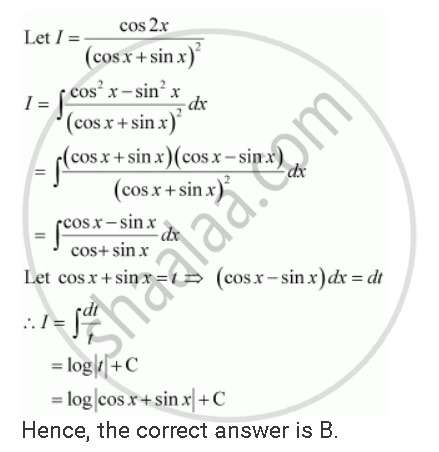Share

# Choose the Correct Answers Int (Cos 2x)/(Sin X + Cos X)^2dx is Equal to - CBSE (Science) Class 12 - Mathematics

ConceptDefinite Integral as the Limit of a Sum

#### Question

Choose the correct answers int (cos 2x)/(sin x + cos x)^2dx is equal to

A (-1)/(sin x + cos x) + C

(B) log |sin x + cos x | + C

(B) log |sin x + cos x | + C

(D) 1/(sin x - cos x)^2

#### SolutionIs there an error in this question or solution?

#### Video TutorialsVIEW ALL 

Solution Choose the Correct Answers Int (Cos 2x)/(Sin X + Cos X)^2dx is Equal to Concept: Definite Integral as the Limit of a Sum.
S